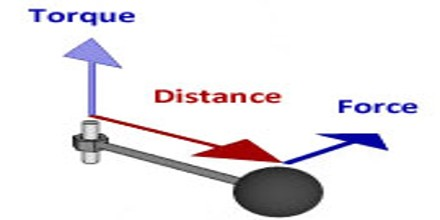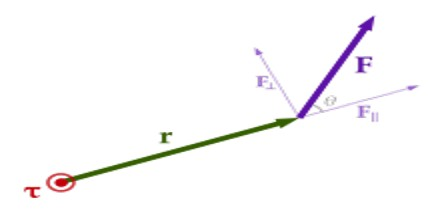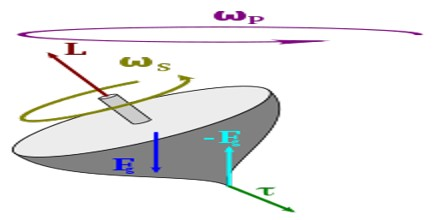Torque

Definition

Torque is the tendency of a force applied to an object to make it rotate about an axis. It is also a vector quantity, equal to the vector product of the vector pointing from the axis to the point of application of force and the vector of force; torque thus points upward from a counterclockwise rotation.

Torque is a measure of the turning force on an object such as a bolt or a flywheel. For example, pushing or pulling the handle of a wrench connected to a nut or bolt produces a torque (turning force) that loosens or tightens the nut or bolt. The symbol for torque is typically Τ, the lowercase Greek letter tau. When it is called moment of Τforce, it is commonly denoted by M.The concept of torque, also called moment or couple, originated with the studies of Archimedes on levers. The term torque was apparently introduced into English scientific literature by James Thomson, the brother of Lord Kelvin, in 1884.

Torque is defined as a twisting force that tends to cause rotation. We call the point where the object rotates the axis of rotation. We use torque every day without realizing it. We apply torque three times when we simply open a locked door. Turning the key, turning the doorknob, and pushing the door open so it swings on its hinges are all methods of applying a torque.

Torque Calculation

Torque is the result of multiplying the value of Force applied by the Distance from the point of application It should also be realised that some Torque Wrenches are length dependent which means that the actual torque applied to the fastener varies if the hand position on the wrench is varied – even with the wrench preset. This occurs if the pivot point of the wrench mechanism is not coincidental with the point of application of torque.The magnitude of the torque vector τ for a torque produced by a given force F is

τ = F . r sin(θ)

Where r is the length of the moment arm and θ is the angle between the force vector and the moment arm. In the case of the door shown, the force is at right angles (90) to the moment arm, so the sine term becomes 1 and τ =Fr.

The direction of the torque vector is found by convention using the right hand grip rule. If a hand is curled around the axis of rotation with the fingers pointing in the direction of the force.

Physics of Torque

In order to find a linear force we need to know a mass and acceleration. However, torque is little different, thanks to rotation being involved. We need to know not only the mass and acceleration of a linear force, but also how far that force is from the axis of rotation, since we can get different results depending on that, as well. We can see this in the diagram and equation for torque.T = F * r * sin(θ)

T = torque

F = linear force

r = distance measured from the axis of rotation to where the linear force is applied

theta = the angle between F and r

In our equation, sin(θ) has no units, r has units of meters (m), and F has units of Newtons (N). Combining these together, we can see that a unit of torque is a Newton-meter (Nm).

Finally, theta is needed to take into account the direction from which the linear force is being applied. The force will not always be pushed from straight on like a door. It can come from many different angles.

Torque is part of the basic specification of an engine: the power output of an engine is expressed as its torque multiplied by its rotational speed of the axis. Internal-combustion engines produce useful torque only over a limited range of rotational speeds (typically from around 1,000–6,000 rpm for a small car). The varying torque output over that range can be measured with a dynamometer, and shown as a torque curve.